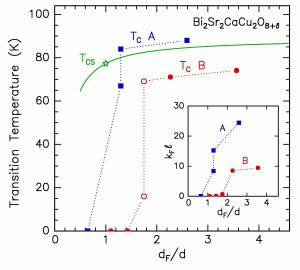# High-Tc Superconductivity in Ultra-thin Crystals: Implications for microscopic theory

High-TC Superconductivity in Ultra-thin Crystals: Implications for microscopic theory, D. R. Harshman and A. T. Fiory [arXiv]
High transition temperature (high-TC) superconductivity is associated with layered crystal structures. This work considers superconductivity in ultra-thin crystals (of thickness equal to the transverse structural periodicity distance d for one formula unit) of thirty-two cuprate, ruthenate, rutheno-cuprate, iron pnictide, organic, and transuranic compounds, wherein intrinsic optimal (highest) transition temperatures TC0 (10 – 150 K) are assumed. Sheet transition temperatures TCS = α TC0, where α < 1, are determined from Kosterlitz-Thouless (KT) theory of phase transitions in two-dimensional superconductors. Calculation of a involves superconducting sheet carrier densities NS derived theoretically from crystal structure, ionic valences, and known doping, a two-fluid model for the temperature dependence of the superconducting magnetic penetration depth, and experimental data on KT transitions; a is on average 0.83 (varying with standard deviation 0.11). Experiments on thin crystal structures approaching thickness d are shown to be consistent with calculations of TC0 from microscopic superconductivity theory and with TCS from KT theory, where the presence of disorder is also taken into account; careful analyses of these thin film studies indicate a minimum thickness dFd for superconductivity.The transition temperature TC and kFℓ (inset), both plotted as a function of dF/d for thin-film Bi2Sr2CaCu2O8+δ samples; The data denoted as “A” (blue squares) correspond to Saito and Kaise (1998) and “B” (red circles) are from Sugimoto et al. (1991). The solid curve is the theoretical calculation of TCS versus dF/d with TC0 = 89 K; the star corresponds to dF/d = 1.

D. R. Harshman and A. T. Fiory, Emerging Materials Research 1, 4 (2012).